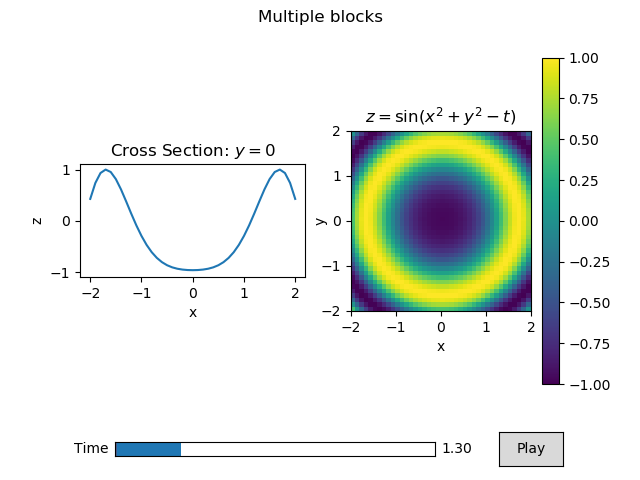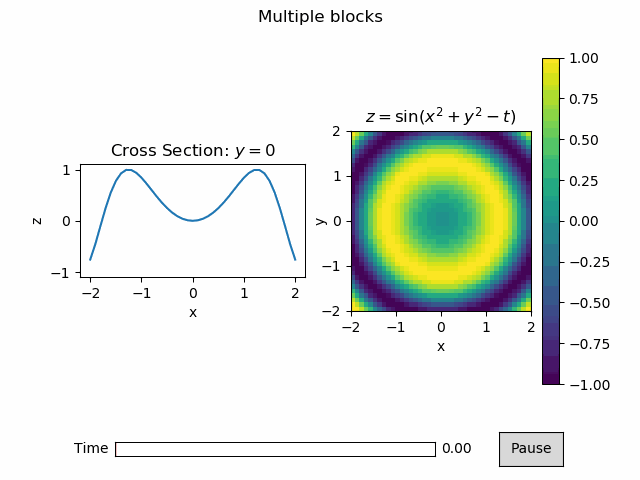# Using multiple blocks¶

Here we are going to use 2 different blocks in our animation.

First we need some imports:

:

%matplotlib notebook
import numpy as np
import matplotlib.pyplot as plt
import animatplot as amp


We are going to plot a pcolormesh and a line on 2 different axes.

Let’s use: $$z = \sin(x^2+y^2-t)$$ for the pcolormesh, and a cross-section of $$y=0$$: $$z = \sin(x^2-t)$$ for the line.

First, we generate the data.

:

x = np.linspace(-2, 2, 41)
y = np.linspace(-2, 2, 41)
t = np.linspace(0, 2*np.pi, 30)

X, Y, T = np.meshgrid(x, y, t)

pcolormesh_data = np.sin(X*X+Y*Y-T)
line_data       = pcolormesh_data[20,:,:] # the slice where y=0


We need to be careful here. Our time axis is the last axis of our data, but animatplot assumes it is the first axis by default. Fortunately, we can use the t_axis argument.

We use the ax argument to attached the data to a specific subplot.

:

# standard matplotlib stuff
# create the different plotting axes
fig, (ax1, ax2) = plt.subplots(1, 2)

for ax in [ax1, ax2]:
ax.set_aspect('equal')
ax.set_xlabel('x')

ax1.set_ylabel('z')
ax1.set_ylim([-1.1,1.1])

fig.suptitle('Multiple blocks')
ax1.set_title('Cross Section: $y=0$')
ax2.set_title(r'$z=\sin(x^2+y^2-t)$')

# animatplot stuff
# now we make our blocks
line_block       = amp.blocks.Line(X[0,:,:], line_data,
ax=ax1, t_axis=1)
pcolormesh_block = amp.blocks.Pcolormesh(X[:,:,0], Y[:,:,0], pcolormesh_data,
ax=ax2, t_axis=2, vmin=-1, vmax=1)
timeline = amp.Timeline(t, fps=10)

# now to contruct the animation
anim = amp.Animation([pcolormesh_block, line_block], timeline)
anim.controls()

anim.save_gif('images/multiblock')
plt.show()There is a lot going on here so lets break it down.

Firstly, the standard matplotlib stuff is creating, and labeling all of our axes for our subplot. This is exactly how one might do a static, non-animated plot.

When we make the Line block, we pass in the data for our lines as 2D arrays (X[0,:,:] and line_data). We attached that line to the first matplotlib axes ax=ax1. We also specifify that the time axis is the last axis of the data t_axis=1.

When we make the Pcolormesh block, we pass in the x, y data as 2D arrays (X[:,:,0] and Y[:,:,0]), and the z data as a 3D array. We attached the pcolormesh to the second matplotlib axes ax=ax2. We also specifify that the time axis is the last axis of the data t_axis=2.

Additional, we told the Pcolormesh blocks what the minimum and maximum values will be (vmin=-1 and vmax=1), so that the colorscale will be proper. The keywords vmin, and vmax get passed to the underlaying called to matplotlib’s pcolormesh.

plt.colorbar does not recognize the Pcolormesh block as a mappable, so we pass in a mappable from the block to get the colorbar to work. In the future, animatplot may have a wrapper around this.

The rest simply brings all of the blocks, and the timeline together into an animation.